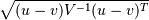# scipy.spatial.distance.mahalanobis¶

scipy.spatial.distance.mahalanobis(u, v, VI)[source]

Computes the Mahalanobis distance between two 1-D arrays.

The Mahalanobis distance between 1-D arrays u and v, is defined aswhere V is the covariance matrix. Note that the argument VI is the inverse of V.

Parameters: u : (N,) array_like Input array. v : (N,) array_like Input array. VI : ndarray The inverse of the covariance matrix. mahalanobis : double The Mahalanobis distance between vectors u and v.

#### Previous topic

scipy.spatial.distance.kulsinski

#### Next topic

scipy.spatial.distance.matching